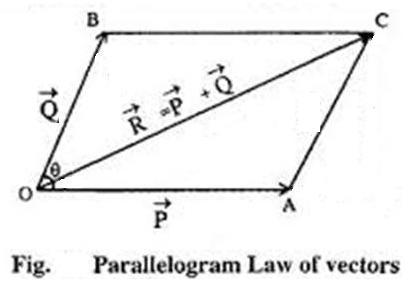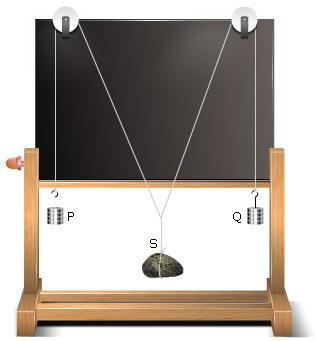Parallelogram Law of Vectors.

# Objective

Our objective is to find the weight of a given body using the Parallelogram Law of Vectors.

# Theory

## What does the Parallelogram Law of Vectors state?If  two vectors acting simultaneously on a particle are represented in magnitude and direction by the two adjacent sides of a parallelogram drawn from a point, then their resultant is completely represented in magnitude and direction by the diagonal of that parallelogram drawn from that point.

## Parallelogram Law of Vectors explained

Let two vectors P and Q act simultaneously on a particle O at an angle. They are represented in magnitude and direction by the adjacent sides OA and OB of a parallelogram OACB drawn from a point O.Then the diagonal OC passing through O, will represent the resultant R in magnitude and direction.

On a Gravesand's apparatus, if the body of unknown weight (say S) is suspended from the middle hanger and balancing weights P and Q are suspended from othe two hangers then,orThe unknown weight can be calculated from the equation (1).

On a Gravesand's apparatus, if the body of unknown weight (say S) is suspended from the middle hanger and balancing weightsP and Q are suspended from the other two hangers then,Now construct a parallelogram OACB by assuming a scale (say 1cm=50 gwt) corresponding to the weights P and Q. The diagonal of the parallelogram OC will give the resultant vector. The weight of the unknown body,If W is the actual weight of the body, then the percentage error in the experiment can be calculated using the equation,## Learning Outcomes

• Students learn what is parallelogram law of vectors.
• They become familiar with the Gravesands apparatus.
• Students are able to find the unknown weight of an object using the parallelogram law of vectors.